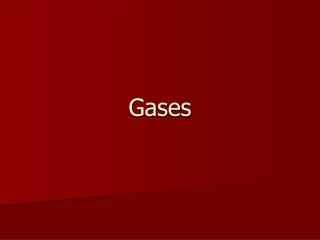DownloadDownload PresentationGases

# Gases

Télécharger la présentation## Gases

- - - - - - - - - - - - - - - - - - - - - - - - - - - E N D - - - - - - - - - - - - - - - - - - - - - - - - - - -
##### Presentation Transcript

1. Gases

2. Characteristics of Gases • Gases are fluids • In other words, they can flow. • Gases have low density • Most of the volume occupied by gases are empty space. • Gases are highly compressible • Completely fills a container

3. Pressure • Pressure is defined as the force divided by area. • So, it is the force on an area. • That is why scientists derived the pascal to measure pressure. • A pascal is defined as one newton (the SI unit for force) applied over an area of one square meter. • Pressure is caused when a gas collides with the walls of a container. • Collisions = force, container walls give us the area over which the force is applied

4. How do we measure pressure? • One way is with a barometer • The most common type use a liquid to measure the atmospheric pressure.

5. How is pressure recorded? • There are several different pressure units that can be used.

6. Example problems Convert each of the following pressures into the specified unit. • 72.7 atm  pascals • 12.33 kPa  millimeters of mercury (hint kPa means 1000 Pa) • 100.0 kPa  atmospheres • 44.7 psi  pascals

7. Answers • 7366327.5 pascals • 92.5 millimeters of mercury • 0.987 atmospheres • 308196 pascals

8. Kinetic Molecular Theory • Book Definition - A theory that explains that the behavior of physical systems depends on the combined actions of the molecules constituting the system. • Another way to say this – the behavior of a system depends on the actions of the molecules that make it up.

9. Concepts of the Kinetic Molecular Theory • All matter is composed of particles. • All matter is in constant motion • Gases particles are in constant, rapid, and random motion • This motion represents the kinetic energy (KE = ½ mv2) of the system. • Particles of a gas are veryfar apart relative to their size. • All collisions are perfectly elastic. In other words, there is no loss or gain in energy.

10. Temperature and Kinetic Energy • The average kinetic energy of random motion is proportional to the absolute temperature (temperature in Kelvin) • So, if the temperature changes; the random motion changes. • If temperature increases; random motion increases. • Note: this does not mean that all particles are moving at the same speed, but overall the rates increase the average.

11. Homework • Page 422: 8, 9, 10 • Page 446: 32, 34